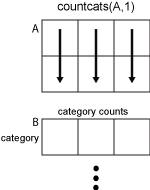# countcats

## 语法

``B = countcats(A)``
``B = countcats(A,dim)``

## 说明

````B = countcats(A)` 返回分类数组 `A` 的每个类别中的元素数。 如果 `A` 是向量，则 `countcats` 返回每个类别中的元素数。如果 `A` 是矩阵，则 `countcats` 将 `A` 的各列视为向量，并返回 `A` 的每列的类别计数。如果 `A` 是多维数组，则 `countcats` 沿大小不等于 `1` 的第一个数组维度计算。 要显示类别名称和每个类别中元素数的分类数组的汇总，请使用 `summary` 函数。```

``B = countcats(A,dim)` 返回沿维度 `dim` 的类别计数。例如，可以使用 `countcats(A,2)` 返回分类数组中每行的类别计数。`

## 示例

`A = categorical({'plane' 'car' 'train' 'car' 'plane'})`
```A = 1x5 categorical plane car train car plane ```

`A` 包含三个类别。要列出类别及其顺序，请使用 `categories` 函数。`A` 的每个元素都属于通过调用 `categories` 所列出的类别之一。

`categories(A)`
```ans = 3x1 cell {'car' } {'plane'} {'train'} ```

`B = countcats(A)`
```B = 1×3 2 2 1 ```

`B` 中的第一个元素对应于 `A` 的第一个类别，即 `car``B` 中的第二个元素对应于 `A` 的第二个类别，即 `plane``B` 中的第三个元素对应于 `A` 的第三个类别，即 `train`

```valueset = 1:3; catnames = {'red' 'green' 'blue'}; A = categorical([1 3; 2 1; 3 1],valueset,catnames)```
```A = 3x2 categorical red blue green red blue red ```

`A` 包含三个类别：`red``green``blue`

`B = countcats(A)`
```B = 3×2 1 2 1 0 1 1 ```

`B` 的第一行对应于 `A` 的第一个类别。值 `red``A` 的第一列中出现一次，在第二列中出现两次。

`B` 的第二行对应于 `A` 的第二个类别。值 `green``A` 的第一列中出现一次，在第二列中不出现。

`B` 的第三行对应于 `A` 的第三个类别。值 `blue``A` 的第一列中出现一次，在第二列中出现一次。

```valueset = 1:3; catnames = {'red' 'green' 'blue'}; A = categorical([1 3; 2 1; 3 1],valueset,catnames)```
```A = 3x2 categorical red blue green red blue red ```

`A` 包含三个类别：`red``green``blue`

`B = countcats(A,2)`
```B = 3×3 1 0 1 1 1 0 1 0 1 ```

`B` 的第一列对应于 `A` 的第一个类别。值 `red``A` 的第一行以及第二行、第三行中出现一次。

`B` 的第二列对应于 `A` 的第二个类别。值 `green` 仅出现在一个元素中。该元素位于 `A` 的第二行中。

`B` 的第三列对应于 `A` 的第三个类别。值 `blue``A` 的第一行中出现一次，在第三行中出现一次。

```valueset = 1:3; catnames = {'red' 'green' 'blue'}; A = categorical([1;3;2;1;3;1],valueset,catnames)```
```A = 6x1 categorical red blue green red blue red ```

`A = removecats(A,'blue')`
```A = 6x1 categorical red <undefined> green red <undefined> red ```

`A` 包含两个类别：`red``green``blue` 中来自 `A` 类别的元素现在未定义。

`B = countcats(A)`
```B = 2×1 3 1 ```

`B` 中的第一个元素对应于 `A` 的第一个类别。值 `red``A` 中出现三次。

`B` 中的第二个元素对应于 `A` 的第二个类别。值 `green``A` 中出现一次。

`countcats` 不返回有关未定义元素的任何信息。

`summary(A)`
``` red 3 green 1 <undefined> 2 ```

## 输入参数## 提示

• 要计算分类数组 `A` 中未定义的元素数，必须使用 `summary``isundefined`